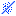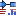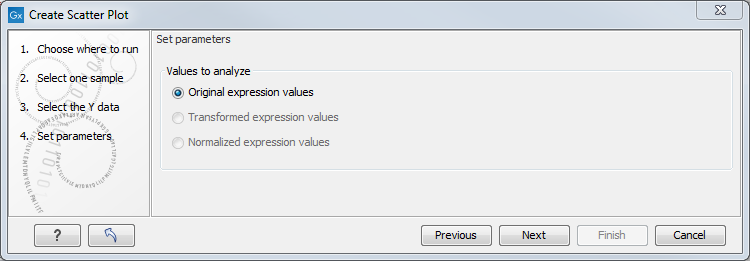## Scatter plot

As described in Scatter plot view of an experiment, an experiment can be viewed as a scatter plot. However, you can also create a "stand-alone" scatter plot of two samples:

Toolbox | Microarray and Small RNA Analysis ()| General Plots | Create Scatter Plot ()

In the first two dialogs, select two samples ( (), () or ()): the first is the sample that will be plotted on the X axis of the plot, the second the one that will define the Y axis. Clicking Next will display a dialog as shown in figure 30.90.Figure 30.90: Selecting which values the scatter plot should be based on.

In this dialog, you select the values to be used for creating the scatter plot (see Selecting transformed and normalized values for analysis).

Click Finish to start the tool.